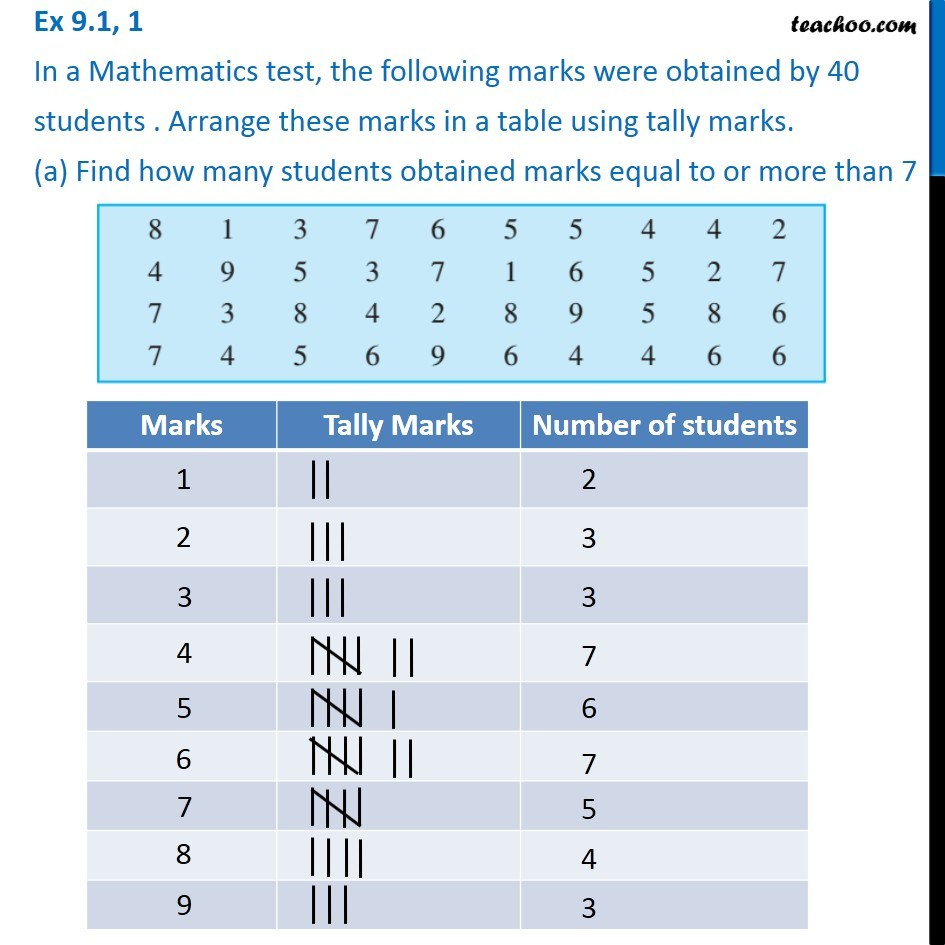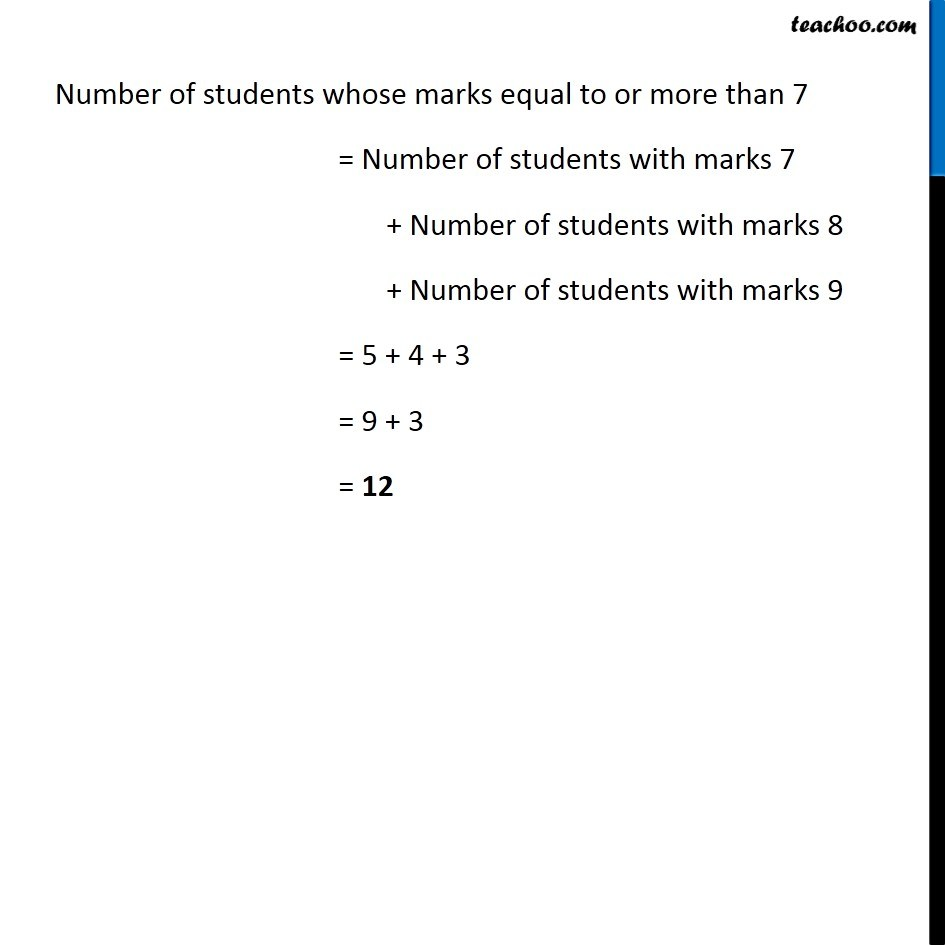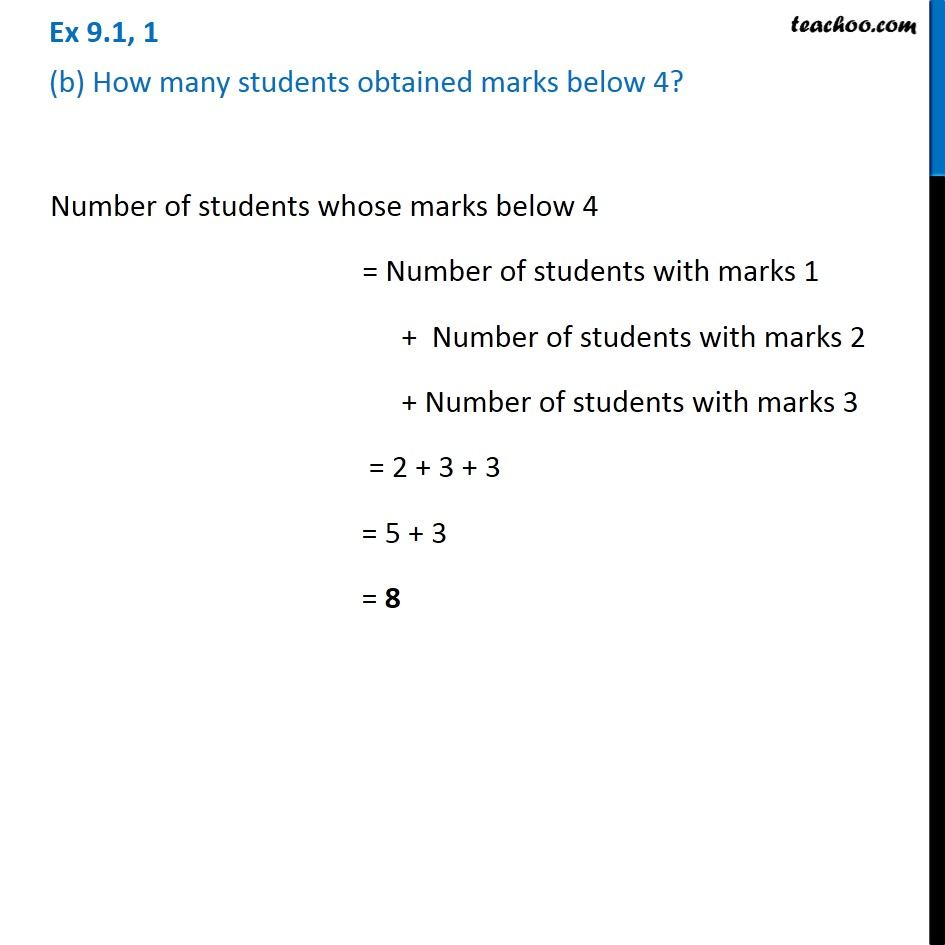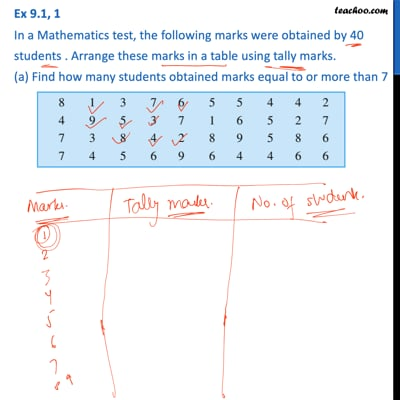Ex 9.1

Chapter 9 Class 6 Data Handling
Serial order wiseThis video is only available for Teachoo black users

### Transcript

Ex 9.1, 1 In a Mathematics test, the following marks were obtained by 40 students . Arrange these marks in a table using tally marks. (a) Find how many students obtained marks equal to or more than 7 Number of students whose marks equal to or more than 7 = Number of students with marks 7 + Number of students with marks 8 + Number of students with marks 9 = 5 + 4 + 3 = 9 + 3 = 12 Ex 9.1, 1 (b) How many students obtained marks below 4?Number of students whose marks below 4 = Number of students with marks 1 + Number of students with marks 2 + Number of students with marks 3 = 2 + 3 + 3 = 5 + 3 = 8